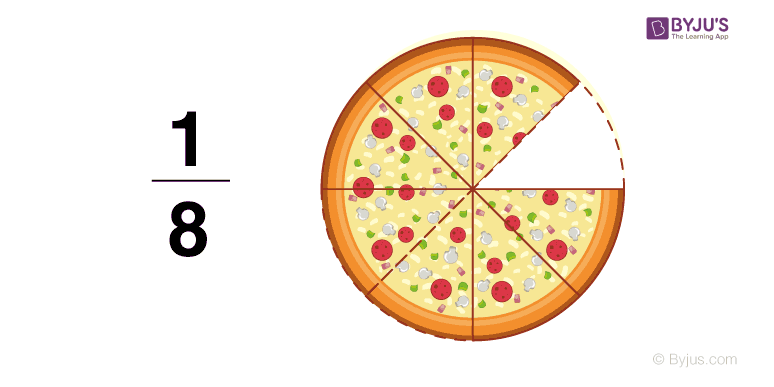# Fractions

A fraction is a part of a thing or a group of things. Fractions are written in the form of a numerator and denominator.

For example:

$$\begin{array}{l} \frac{1}{2} \end{array}$$

Here 1 is the numerator and 2 is the denominator

In fractions, the upper part or the numerator represents the parts out of the total, and the lower part or the denominator is the total parts.In the above given pizza image —

$$\begin{array}{l}\frac{1}{8}\end{array}$$
• 1 is the numerator, which represents a part or one slice of a pizza
• 8 is the denominator, which represents the total slices of a pizza

## Equal or Equivalent Fractions

Multiplying the numerator and denominator of a fraction by the same number (except 0) will not change its value. These fractions are called equal or equivalent.

Example:

$$\begin{array}{l} \frac{1 * 2}{2 * 2} = \frac{2}{4} \end{array}$$

Examples of equal or equivalent fractions:

$$\begin{array}{l}\frac{1}{2} = \frac{2}{4} =\frac{3}{6} =\frac{4}{8} =\frac{5}{10}\end{array}$$
$$\begin{array}{l}\frac{1}{3} = \frac{2}{6} =\frac{3}{9} =\frac{4}{12} =\frac{5}{15} \end{array}$$
$$\begin{array}{l}\frac{1}{4} = \frac{2}{8} =\frac{3}{12} =\frac{4}{16} =\frac{5}{20} \end{array}$$

How to convert fractions into equivalent fractions.

If two fractions are equivalent, then the product of the numerator of one fraction with the other fraction’s denominator will be equal.

For example:

$$\begin{array}{l}\frac{25}{45} = \frac{5}{9} \end{array}$$

The denominator of the second fraction is 5 times the denominator of the first fraction. The numerator of the second fraction will also be 5 times the numerator of the first fraction. Unknown numerator = 2 * 5 = 10

In the below given example, the fractions have different numerators and denominators but are equal to the same value.

25 * 9 = 225

45 * 5 = 225

$$\begin{array}{l}\frac{25}{45} = \frac{5}{9} \end{array}$$

Therefore, it is an equivalent fraction.

### Like Fraction

The fractions with equal denominators are called like fractions.

Example:

$$\begin{array}{l} \frac{1}{10}, \frac{2}{10}, \frac{3}{10}, \frac{4}{10},\frac{5}{10} \end{array}$$

### Proper, Improper and Mixed Fraction

The fractions with smaller numerators are called proper fractions.

Example:

$$\begin{array}{l} \frac{1}{2}, \frac{1}{4}, \frac{1}{5}, \frac{2}{3}, \frac{2}{7}\end{array}$$

The fractions with greater or equal numerators are called improper fractions.

Example:

$$\begin{array}{l} \frac{5}{3}, \frac{5}{4}, \frac{3}{3}, \frac{7}{5}, \frac{4}{3} \end{array}$$

Writing an improper fraction in the form of a whole number and a fractional part is called a mixed fraction.

Example:

$$\begin{array}{l} 1\frac{2}{5}, 1\frac{2}{7},3\frac{1}{3}, 3\frac{1}{4}, 5\frac{1}{2} \end{array}$$

Stay tuned to BYJU’S for more information on NIOS, syllabus, notes, along with its important questions and solutions.